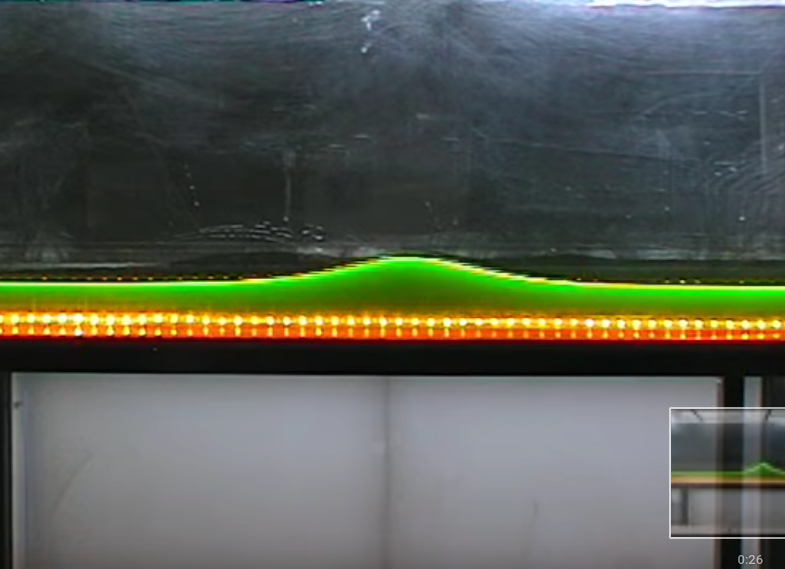# What is… an integrable system?

The oversimplified answer is that integrable systems are equations with a lot of structure. The kind of equations we are thinking about are differential equations, which describe change…The oversimplified answer is that integrable systems are equations with a lot of structure.

The kind of equations we are thinking about are differential equations, which describe change. Whenever something is moving, you can count on it that physicists would like to describe it using differential equations. It could be an apple falling from a tree or the earth orbiting the sun, the pendulum of a grandfather clock or the waves carrying your phone signal. Here, we’ll look at water waves.

## Playing in the water

Let’s start by throwing a pebble in a pond. If you look carefully at the waves it creates, you’ll see some interesting things. You might observe, for example, that longer waves travel faster than shorter waves. After a while you’ll see a sequence of circular waves that are shorter on the inside and longer on the outside:

This effect, that the speed of a wave depends on the wavelength, is called dispersion. The wake of a boat provides another example:

A second effect that you can observe in the videos above is that the individual wave crests and troughs do not travel at the same speed as the big pattern. The velocity of the individual waves is called the phase velocity, the velocity of the whole pattern is called the group velocity.

These examples show typical behaviour of waves. The wave fronts change shape and are torn apart. Compared to the ocean on a stormy day, the waves we’ve seen so far are quite tame, but still things get complicated if we try to understand the details.

## Integrability

What we saw above is the way most waves work, but they are not integrable systems. Integrable systems are the exceptions. They are differential equations with solutions that are easy to understand. Integrable wave equations describe waves that are really quite boring.

The waves in an integrable system preserve their shape:

Such a wave is called a soliton, because it occurs by itself and (to theoretical physicists) looks like an elementary particle (particle names often end in -on).

Waves in a narrow and shallow channel like in this video are described by the Kortweg-de Vries equation. This equation is one of the most important examples of an integrable wave equation. The understanding of the Korteweg-de Vries equation as an integrable system dates mostly to the 1960s and 70s, but its history started over a century earlier with a Victorian engineer on horseback chasing a soliton along Scottish canal.

## Hidden mathematical structure

Unfortunately “having well-behaved solutions” is not a very good mathematical definition of an integrable system. We want to know the underlying reason why certain equations have nice solution. The explanation is usually some hidden structure.

One type of hidden structure that can make a system integrable is the existence of a large amount of conserved quantities. Like its name suggests, a conserved quantity is something that does not change in time. Most systems in physics have conserve quantities like energy and (angular) momentum, but not many more than those. Integrable systems are those that do have a large number of conserved quantities.

In a way, each conserved quantity is an extra constraint that the system must satisfy: the system must always preserve this quantity. So if there are many conserved quantities, then there are many constraints a solution must fulfil. The Kortweg-de Vries equation has an infinite amount of conserved quantities. Therefore, there are infinitely many constraints that the wave must satisfy. This leaves it with little freedom to change its shape. Initially there might still be some complicated things going on, with smaller waves behaving erratically, but once a large stable wave is formed, it will keep its shape forever.

Conserved quantities are not only part of the explanation of the nice qualitative behaviour we observed, they also help to make quantitative statements about a system. Knowing conserved quantities is very helpful to derive exact solutions of a differential equation. An exact solution is a formula that tells you precisely which shape and position the wave takes at each instant in time. Nowadays we call finding exact solutions “solving” a differential equation, but in the 19th century people would say “integrating” instead. This is where the name integrable system comes from.

## Soliton interaction

When two solitons collide, weird stuff happens. Their interaction is complicated and looks a bit chaotic. You’d expect the combined wave to be taller, but actually the height of the crest decreases during the interaction.

But then, magic! After the interaction, the two original waves appear again, as if nothing at all has happened! Even though the waves seem to change during the interaction, the integrable system “remembers” everything about them and in the end they are restored.

## One sentence about my work

My research involves a relatively new approach to integrable systems, which is to describe them using variational principles.

You can find out here what a variational principle is. Or, if you’ve had enough maths for one day, you can head to Gloucestershire instead and try to surf a soliton:

You can find a more technical introduction
to integrable systems in these slides